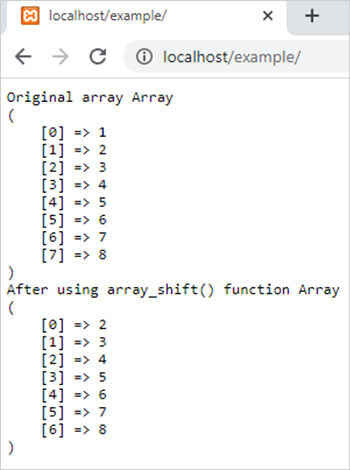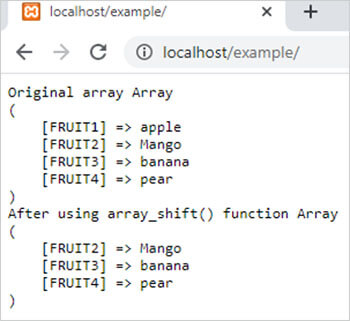array_shift() function in PHP || PHP Array Shift Example

# array_shift() function in php

0 1151

array_shift() function of PHP used to shift the elements of array one position in left means it removes the initial or starting element of an array. If the array is an index array then it re-indexed the array from 0.

Syntax:

array_shift(\$array)

here,

\$array is a PHP array.

Example 1:

```<?php
\$arr1=array(1,2,3,4,5,6,7,8);
echo "<pre>";
echo "Original array ";
print_r(\$arr1);
array_shift(\$arr1);
echo "After using array_shift() function ";
print_r(\$arr1);
?>```

Output:Example 2:

```<?php
\$arr1=array("FRUIT1"=>"apple","FRUIT2"=>"Mango","FRUIT3"=>"banana","FRUIT4"=>"pear");
echo "<pre>";
echo "Original array";
print_r(\$arr1);
array_shift(\$arr1);
echo "After using array_shift() function";
print_r(\$arr1);
?>```

Output:Share: